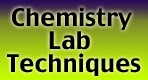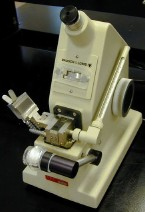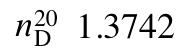## Refractometry:A Bausch & Lomb Abbe-3L Refractometer.

## Introduction

A refractometer measures the extent to which light is bent (i.e. refracted) when it moves from air into a sample and is typically used to determine the index of refraction (aka refractive index or n) of a liquid sample.

The refractive index is a unitless number, between 1.3000 and 1.7000 for most compounds, and is normally determined to five digit precision. Since the index of refraction depends on both the temperature of the sample and the wavelength of light used these are both indicated when reporting the refractive index:The italicized n denotes refractive index, the superscript indicates the temperature in degrees Celsius, and the subscript denotes the wavelength of light (in this case the D indicates the sodium D line at 589 nm).

The refractive index is commonly determined as part of the characterization of liquid samples, in much the same way that melting points are routinely obtained to characterize solid compounds. It is also commonly used to:

• Help identify or confirm the identity of a sample by comparing its refractive index to known values.
• Assess the purity of a sample by comparing its refractive index to the value for the pure substance.
• Determine the concentration of a solute in a solution by comparing the solution's refractive index to a standard curve.